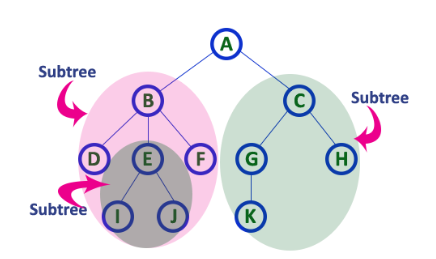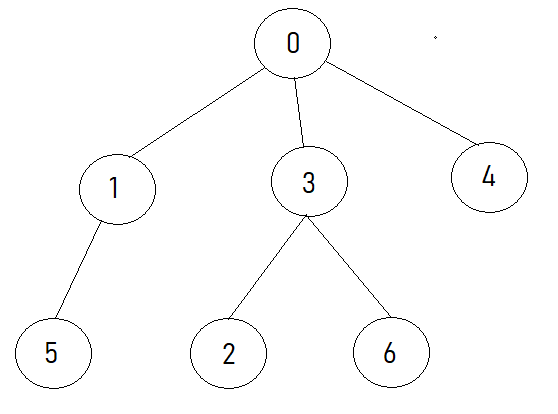2

# Count elements in all subtrees

Difficulty: EASY
Avg. time to solve
10 min
Success Rate
90%

Problem Statement
Suggest Edit

#### You are given an arbitrary tree consisting of N nodes numbered from 0 to N-1. You need to find the total number of elements in all the subtrees of the given tree. In other words, given a generic tree, find the count of elements in the subtrees rooted at each node.

``````A subtree of a tree T is a tree S consisting of a node in T and all of its descendants in T. The subtree corresponding to the root node is the entire tree. For better understanding, refer to the image below:-
``````##### Note:
``````1. The tree will always be rooted at 0.
2. You can return the count of nodes in any order.
3. The root of any subtree is also counted in the subtree thus the count of nodes in a subtree rooted at a leaf node is
4. You can return the numbers in any order.
``````
##### Input Format:
``````The first line of the input contains a single integer T, representing the number of test cases.
The first line of each test case consists of a single integer N, representing the number of nodes in the given tree.
The next N-1 lines of each test case contain two space-separated integers u and v, denoting an edge between the node u and the node v.
``````
##### Output Format:
``````For each test case, print the number of nodes in all subtrees of the given tree, in any order.
``````
##### Note:
``````You do not need to print anything. It has already been taken care of. Just implement the given function.
``````
##### Constraints:
``````1 <= T <= 100
1 <= N, E <= 10^4
0 <= U,V < N
Time Limit: 1sec
``````
##### Sample Input 1:
``````1
7
0 1
0 3
0 4
1 5
3 2
3 6
``````
##### Sample Output 1:
``````7 2 1 3 1 1 1
``````
##### Explanation for sample input 1:
``````The above tree looks like -
````````````The subtrees rooted at 5, 2, 6, 4 have 1 node each.
The subtree rooted at 1 has 2 nodes.
The subtree rooted at 3 has 3 nodes.
The subtree rooted at 0 has 7 nodes.
Hence, the output is {1, 1, 1, 1, 2, 3, 7}.
``````
##### Sample Input 2:
``````1
3
0 1
0 2
``````
##### Sample Output 2:
``````1 1 3
``````